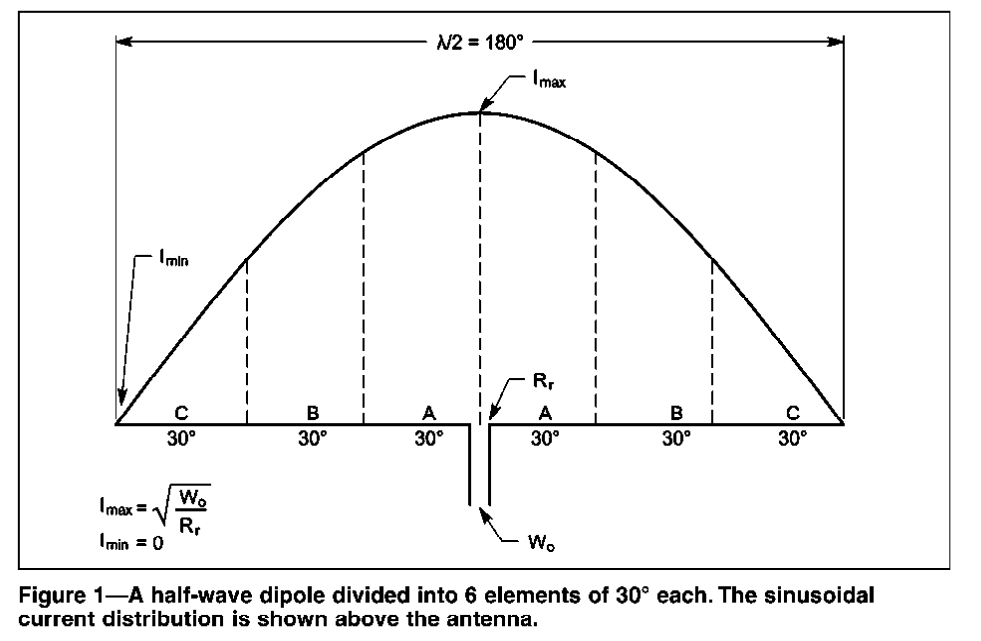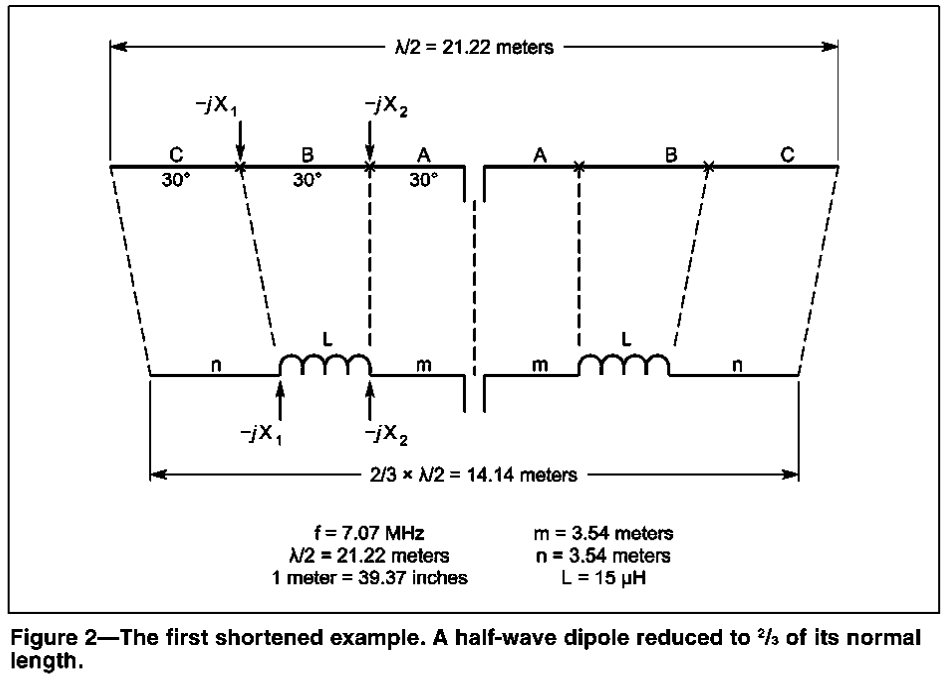# test html 2

– no title specified

 How to shorten a Dipole AntennaHalf Wave  = 180 degrees Quarter Wave = 90 degrees Section B is  the antenna section to be shortened by using an inductor. The -j X1 and -jX2 represent capacitance for the section being shortened.   In order to do this an inductor is made which cancels the capacitance for the section B. Two formulas are used for determining the inductor value: X = -jZo Co-tangent(Beta) or x= -j*Zo*cot(Beta) Zo = 138 * log(4*h/d) h is the height of the antenna above ground in meters. d is the wire diameter used to make the inductor in millimeters. -JX1= -j*Zo(Cot(X1 in degrees)) -JX2= -j*Zo(Cot(X2 in degrees)) XL= -JX2 -(-JX1) => XL = jX1 – JX2 X1 = -j*Zo*(Cot(X1 in degrees)) X2= -j*Zo*(Cot(X2 in degrees)) X1 is greater than X2 Calulations: Resonance_Freq 7.07 Mhz Wire_Length 21.2164073550212 Meters 69.5898161244696 Ft 0.110508628005658 Resistance 12 Gauge Wire 0.001588 Ohms/ft Height of Antenna 34.7949080622348 in Feet 1/2 wave length at feedpoint Wire Diameter 2 millimeter Zo 597.065369926047 Ohms X1 angle 30 Degrees n length 3.53606789250354 in meters 0.005615275813296 Resistance X2 delta angle 30 Degrees m length 3.53606789250354 in meters 0.005615275813296 Resistance Inductor Calculations -JX1 1034.14755615182 Ohms a 2 Form Diameter in inches -jX2 344.715852050607 Ohms b 4 Form length in inches n 27 Number of Turns jXL 689.431704101214 Ohms 339.292006587698 wire length 1.07759141292253 1.08882196454912 Coil Inductance 15.5200090002796 uH Inductor 14.8775510204082 uH Antenna Length 14.1442715700141 meters Center Spacing in ~ 3 inches between the two elements Rr 50 Radiation resistance Rf 1.08882196454912 Ohms Equivalence Resitance of all losses n 97.8687667425476 % Antenna system  efficiency